• 394.0388.0389.0388.0388.0392.0393.0395.0395.0394.0394.0390.0392.0按照之前所了解的，对离散值求导其实就是差分，例如第i点的导数(差分)为：即在一个宽度为2m+1的窗口内通过计算前后m个值加权后的和得到。...
有一组4096长度的数据，需要找到一阶导数从正到负的点，和三阶导数从负到正的点，截取了一小段。394.0388.0389.0388.0388.0392.0393.0395.0395.0394.0394.0390.0392.0按照之前所了解的，对离散值求导其实就是求差分，例如第i点的导数(差分)为：即在一个宽度为2m+1的窗口内通过计算前后m个值加权后的和得到。但是在实际使用过程中效果不是很好。于是想到了同样在一个宽度为2k+1的窗口内，将这2k+1个点拟合成一个函数，然后求导就可以得到任意阶数的导数值。首先是函数拟合，使用from scipy.optimize import leastsq即最小二乘拟合from scipy.optimize import leastsqclass search(object):def __init__(self, filename):self.filename = filenamedef func(self, x, p):f = np.poly1d(p)return f(x)def residuals(self, p, x, y, reg):regularization = 0.1 # 正则化系数lambdaret = y - self.func(x, p)if reg == 1:ret = np.append(ret, np.sqrt(regularization) * p)return retdef LeastSquare(self, data, k=100, order=4, reg=1, show=1): # k为求导窗口宽度,order为多项式阶数,reg为是否正则化l = self.lenstep = 2 * k + 1p =  * orderfor i in range(0, l, step):if i + step < l:y = data[i:i + step]x = np.arange(i, i + step)else:y = data[i:]x = np.arange(i, l)try:r = leastsq(self.residuals, p, args=(x, y, reg))except:print("Error - curve_fit failed")fun = np.poly1d(r) # 返回拟合方程系数df_1 = np.poly1d.deriv(fun) # 求得导函数df_2 = np.poly1d.deriv(df_1)df_3 = np.poly1d.deriv(df_2)df_value = df_1(x)df3_value = df_3(x)fun = np.poly1d(r),fun返回的是一个 polynomial class，具体使用可以见官方文档numpy.poly1dpolynomial对象可以使用deriv方法求导数，求得的依然是 polynomial对象。 df_value = df_1(x)所得到的就是x这个几个点求得的导数值。看似大功告成，但是求导的结果并不是很好，如下图，实际最高点在100左右，但是拟合出来的曲线最高点在120左右，而原因在于使用多项式拟合很难准确拟合曲线。于是想用高斯函数来实现对曲线的拟合，在matlab中试了下，三阶高斯拟合可以很好的拟合曲线，但是numpy以及sicpy中没有找到类似poly1d这种对象，虽然可以自己定义高斯函数，如下def gaussian(self, x, *param):fun = param*np.exp(-np.power(x - param, 2.) / (2 * np.power(param,    2.)))+param*np.exp(-np.power(x - param, 2.) / (2 * np.power(param, 2.)))return fun但是，在通过最小二乘拟合得到函数参数后只能得到拟合后的点，无法直接求导数..所以并不适合。所以还是只能回到多项式拟合，如果4阶多项式不能表征的话，更高阶的呢总体来说，效果还是可以接受的。如果下阶段找到好的高斯函数拟合方法，会继续更新。以上这篇Python求离散序列导数的示例就是小编分享给大家的全部内容了，希望能给大家一个参考，也希望大家多多支持脚本之家。
展开全文• #求函数的导数 import os import numpy as np #定义一个函数y=x**2 def y(x): return x**2 def derivative(x0,deltaX):#x0为所求点横坐标,deltaX为偏移量 return (y(x0+deltaX)-y(x0))/deltaX
求自定义函数的导数
今天我们来讲一下，使用python求自定义函数求倒数的程序
对于这个程序，我们的原理来自于导数的计算公式如下：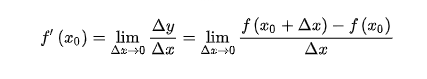那么根据如上公式，我们得到代码如下：
#求函数的导数
import os
import numpy as np
#定义一个函数y=x**2

def  y(x):
return x**2

def  derivative(x0,deltaX):#x0为所求点横坐标,deltaX为偏移量
return (y(x0+deltaX)-y(x0))/deltaX
der=derivative(2,0.1**10)
print("对于y=x**2 在 2处的导数为：", der)
os.system("pause")

最后，我们可以自行修改y()函数，来定义我们想要求解函数的导数
注：该结果求解的导数是近似解


展开全文算法
• 利用python函数求导数的方法：首先python运行环境，导入微分模块包；然后定义符号变量和函数；接着求导【diff = diff(f,x)】；**后输入diff就显示其变量值了。fuG少儿编程网-https://www.pxcodes.comfuG少儿编程网...
利用python函数求导数的方法：首先python运行环境，导入微分的模块包；然后定义符号变量和函数；接着求导【diff = diff(f,x)】；**后输入diff就显示其变量值了。fuG少儿编程网-https://www.pxcodes.comfuG少儿编程网-https://www.pxcodes.com本教程操作环境：windows7系统、python3.9版，DELL G3电脑。fuG少儿编程网-https://www.pxcodes.com利用python函数求导数的方法：fuG少儿编程网-https://www.pxcodes.com1、打开python运行环境fuG少儿编程网-https://www.pxcodes.com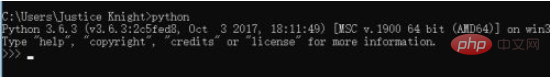fuG少儿编程网-https://www.pxcodes.com2、导入微分的模块包from sympy import *fuG少儿编程网-https://www.pxcodes.com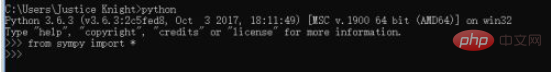fuG少儿编程网-https://www.pxcodes.com3、定义符号变量x = symbols('x')fuG少儿编程网-https://www.pxcodes.comfuG少儿编程网-https://www.pxcodes.com4、定义一个函数f = x**9fuG少儿编程网-https://www.pxcodes.com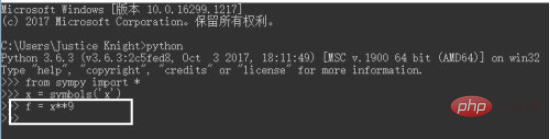fuG少儿编程网-https://www.pxcodes.com5、diff = diff(f,x)求导fuG少儿编程网-https://www.pxcodes.com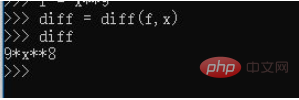fuG少儿编程网-https://www.pxcodes.com6、**后输入diff就显示其变量值了fuG少儿编程网-https://www.pxcodes.com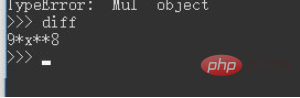fuG少儿编程网-https://www.pxcodes.com相关免费学习推荐：python视频教程fuG少儿编程网-https://www.pxcodes.com
展开全文• 按照之前所了解的，对离散值求导其实就是差分，例如第i点的导数（差分）为： 即在一个宽度为2m+1的窗口内通过计算前后m个值加权后的和得到。但是在实际使用过程中效果不是很好。于是想到了同样在一个宽度为2k+1的...
• 我在pytorch中实现了以下Jacobian函数。除非我犯了错误，否则它计算任何张量w.r.t.雅可比。任何维输入：import torchimport torch.autograd as agdef nd_range(stop, dims = None):if dims == None:dims = len...
我在pytorch中实现了以下Jacobian函数。除非我犯了错误，否则它计算任何张量w.r.t.的雅可比。任何维输入：import torchimport torch.autograd as agdef nd_range(stop, dims = None):if dims == None:dims = len(stop)if not dims:yield ()returnfor outer in nd_range(stop, dims - 1):for inner in range(stop[dims - 1]):yield outer + (inner,)def full_jacobian(f, wrt):f_shape = list(f.size())wrt_shape = list(wrt.size())fs = []f_range = nd_range(f_shape)wrt_range = nd_range(wrt_shape)for f_ind in f_range:grad = ag.grad(f[tuple(f_ind)], wrt, retain_graph=True, create_graph=True)for i in range(len(f_shape)):grad = grad.unsqueeze(0)fs.append(grad)fj = torch.cat(fs, dim=0)fj = fj.view(f_shape + wrt_shape)return fj除此之外，我还尝试实现一个递归函数来计算n阶导数：^{pr2}$我做了一个简单的测试：op = torch.ger(s, s)deep_deriv = nth_derivative(op, s, 5)不幸的是，这成功地得到了Hessian…但是没有高阶导数。我知道很多高阶导数应该是0，但我更希望Pythorch能解析地计算出来。在一个解决办法是将坡度计算改为：try:grad = ag.grad(f[tuple(f_ind)], wrt, retain_graph=True, create_graph=True)except:grad = torch.zeros_like(wrt)这是公认的正确处理方法吗？还是有更好的选择？还是说我的问题一开始就完全错了？在 展开全文• 假设您有两个变量：x = tf.Variable(np.random.random_sample(), dtype=tf.float32)y = tf.Variable(np.random.random_sample(), dtype=tf.float32)以及使用这两个变量定义的函数：f = tf.pow(x, cons(2)) + cons(2)... • 假设有两个变量：x = tf.Variable(np.random.random_sample(), dtype=tf.float32)y = tf.Variable(np.random.random_sample(), dtype=tf.float32)以及使用这两个变量定义的函数：f = tf.pow(x, cons(2)) + cons(2) *... • 当然，在另一个答案中给出的subs选项可用于计算某个数的导数，但如果要绘制导数，则不起作用。有一种方法可以解决这个问题：lambdify，如下所述。使用lambdify将所有sympy函数（可以区分但不能计算）转换为它们的... • 展开全部设参数方程 x(t), y(t)，则二阶导数：一阶...连续函数的一阶导数就是相应的切线斜率。一阶导数大于0，则递增；一阶导数小于0，则递减；一阶导数等于0，则不增不减。而二阶导数可以反映图像的凹凸。... • 在matlab中求解代码：>> syms w;F(w)=8*exp(-j*5*w)/(16+w^2);diff(F(w),w,2)ans =- (200*exp(-w*5*i))/(w^2 + 16) - (16*exp(-w*5*i))/(w^2 + 16)^2 + (64*w^2*exp(-w*5*i))/(w^2 + 16)^3 + (w*exp(-w*5*i)... • 例：函数的导数 f(x)=cos(x)f(x)=cos(x)f(x)=cos(x) 程序，如下 from sympy import * x = symbols('x') print(diff(cos(x), x)) 结果：-sin(x) ~ 2.二阶导数 例：该函数的二阶导数 f(x)=cos2(x)f(x)=cos... • 前面研究了极值存在条件，不知你们是怎么完成，我们接着研究这道题，【解析】函数有极值，则导数 有变号零点.转化为方程 有解问题，观察函数 与 有交点,由直线和 都过定点 ，只要他们不在相切，则必定相交.而... • 2019独角兽企业重金招聘Python工程师标准>>> ... • 精华博览8年新课标I、5年新课标II、4年新课标III高考数学真题详细解析16年新课标I、9年新课标II、4年新课标III高考数学真题分类详解2020年高考数学重要专题讲座2020届全国各地高考数学模拟试题选椭圆与双曲线性质... • 您有四个选项有限差异不需要外部工具，但容易出现数字错误，如果您处于多...看看autowrap或lambdify函数或查看Jensen’s blogpost about a similar question。自动衍生品非常酷，不容易出现数字错误，但需要一些额... • 在要获得导数的精确近似值，需要使h相当小。但是，如果将其设置得太小，那么实际上会由于Python浮点精度有限而失去准确性。使用decimal模块可以获得更高精度。但是，该模块只支持简单算术运算符和平方根函数。... • 机器学习使用线性回归方法建模时，损失函数最优解需要用到最小二乘法。相信很多朋友跟我一样，想先知道公式是什么，然后再研究它是怎么来。所以不多说，先上公式。对于线性回归方程$f(x) = ax + b\$，由最小...
• import scipy from math import sin,cos,exp from sympy import * x=Symbol('x') y=Symbol('y') #求导函数及导数 z1=x**3 ...diff(z1,x).subs(x,5) #在x=5处的导数 即 75 #偏导函数及偏导数 ...偏导数 Symbol subs
• 本文始发于个人公众号：TechFlow，原创不易，个关注今天是Python专题第12篇文章，我们来看看Python装饰器。一段囧事差不多五年前面试时候，我就领教过它重要性。那时候我Python刚刚初学乍练，看完了廖雪峰...
• 我在Keras和TensorFlow一起工作.我有一个深度神经模型(预测自动编码...为此,我需要找到关于特定层输出损失(L)二阶导数(Hessian)： 对角线条目就足够了. L是标量,s是1乘n.我先尝试了什么：dLds = tf.gradients(L,...
• python实现函数求导方法是：1、利用sympy库中symbols方法传入x和y变量；2、利用sympy库中diff函数传入需要求导...需要对一个函数表达式偏导，并将具体数值代入导数式。而python中通常可用于函数求导的函数...
• 例1：下列函数的导数 例2：下列函数的50阶导数 代码如下： from sympy import * x = symbols('x') f1 = diff(tan(x)) f2 = diff(x ** 2 * cos(x), x, 50) print(f1) print(f2) 运行结果： 2.多元...
• 而不是:def function(init, time, k):你应该拥有def function(state, time, k):自从state根据函数返回的导数进行演化。它将只在第一个时间步骤中具有初始值。第三:你的国家解释和国家三角洲是不一致的。...
• python实现函数求导方法是：1、利用sympy库中symbols方法传入x和y变量；2、利用sympy库中diff函数传入需要求导...需要对一个函数表达式偏导，并将具体数值代入导数式。而python中通常可用于函数求导的函数...
• python实现函数求导方法是：1、利用sympy库中symbols方法传入x和y变量；2、利用sympy库中diff函数传入需要求导...需要对一个函数表达式偏导，并将具体数值代入导数式。而python中通常可用于函数求导的函数......

# python求函数的导数python 订阅